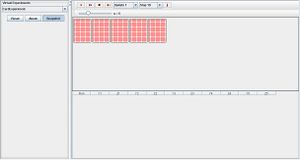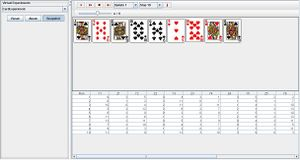# SOCR EduMaterials Activities CardExperiment

## Description

The card experiment demonstrates the basic properties of dealing n cards at random from a regular deck of 52 cards.

Every trial is recorded in the data table below. There are two values for every card, denomination Yi and suit Zi of the i’th card chosen where i= 1, ..., n. The denominations have an interval from 1 to 13 where 1 represents ace, 11 as jack, 12 as queen, and 13 as king. The suits are either 0 (clubs), 1(diamonds), 2(hearts), or 3(spades). The parameter n can be varied with the scroll bar from above. See also these poker game probability calculations.

## Goal

To give an accessible method that explores the characteristics of random conditional probability (e.g. find the probability of selecting a red sock from the sock drawer, a black pen from the pencil box, a plastic cup in the kitchen, etc.) and enforce the knowledge of these events.

## Experiment

Go to the SOCR Experiments and select the Card Experiment from the drop-down list of experiments on the top left. The image below shows the initial view of this experiment:When pressing the play button, one trial will be executed and recorded in the distribution table below. The fast forward button symbolizes the nth number of trials to be executed each time. The stop button ceases any activity and is helpful when the experimenter chooses “continuous,” indicating an infinite number of events. The fourth button will reset the entire experiment, deleting all previous information and data collected. The “update” scroll indicates nth number of trials (1, 10, 100, or 1000) performed when selecting the fast forward button and the “stop” scroll indicates the maximum number of trials in the experiment.

After every card is selected, the probability for a specified card to be chosen will increase. In other words, the probability for a desired card to be chosen first is 1/52. If the card of interest has not been chosen first, then the probability it will be chosen second is 1/51. Again, if the card had not been chosen in the second run, the probability if will be chosen in the third run is 1/50, and so forth. Take note that every trial is ran without replacement.## Applications

For card players, this experiment may be helpful in determining the probability of forming a 5-card hand, pairs, and other types of winning hands. It may also be used for other types of situations:

For example, if a car dealer must randomly select n cars of different horse power and mileage to suit a customer’s satisfaction, then this java applet can illustrate the outcomes of randomly selecting n vehicles within the manufacturer. If the probability of selecting a desirable car for the customer is too low, then the car dealer may then eliminate the types with unfavorable qualities in order to increase the chances of selecting a car that satisfies the customer, thus increasing the sales for the car company.

A restaurant owner is interested in determining the probability of customers selecting an item on the menu. Every entree on the menu has an equal probability of being selected. He uses this applet by noting that the selected item may be on the first, second, third, or fourth page of the menu and if it is located on the top left, top right, bottom left, or bottom right of the page.

## Poker

There is a variety of events that may be of interest for 5-card (poker) games. Some of these are:

• A single Pair Match: For instance, the hand with the pattern AABCD, where A, B, C and D are from the distinct kinds (denominations) of cards: aces, twos, threes, tens, jacks, queens, and kings (there are 13 denominations, and four suits, in the standard 52 card deck). The number of such hands is \({13 \choose 1}*{4\choose 2}*{12\choose 3}*[{4\choose 1}]^3\). If all hands are equally likely, the probability of a single pair is obtained by dividing this number by the total number of 5-card hands possible (\({52\choose 5}=2,598,960\)). Thus, P(1 pair only) = 0.422569.
• Two pairs: For instance, the pattern AABBC where A, B, and C are from distinct kinds. The number of such hands is \({13\choose 2}{4\choose 2}{4\choose 2}{11\choose 1}{4\choose 1}\). And therefore, dividing by (\({52\choose 5}=2,598,960\), the P(2 pairs) = 0.047539.
• One triple: For example, the pattern AAABC where A, B, and C are from distinct kinds. The number of such hands is \({13\choose 1}{4\choose 3}{12\choose 2}{4\choose 1}^2\). Thus, the P(1 triple) = 0.021128.
• A Full House: This includes patterns like AAABB where A and B are from distinct kinds. The number of such hands is \({13\choose 1}{4\choose 3}{12\choose 1}{4\choose 2}\). Thus, P(Full House)=0.001441.
• Four of a kind: For instance, the pattern AAAAB where A and B are from distinct kinds. The number of such hands is \({13\choose 1}{4\choose 4}{12\choose 1}{4\choose 1}\). The P(4-of-a-kind)=0.000240.
• A straight: Five cards in a sequence (e.g., 4,5,6,7,8), with aces allowed to be either 1 or 13 (low or high) and with the cards allowed to be of the same suit (e.g., all hearts) or from some different suits. The number of such hands is \(10*{4\choose 1}^5\). Thus, P(A Straight)=0.003940. But if you exclude Straight-Flushes AND Royal Flushes, the number of such hands is \(10*{4\choose 1}^5 - 36 - 4 = 10200\), the corresponding probability P(A Straight, but not a Straight or Royal Flush)=0.00392465.
• A Straight Flush: All 5 cards are from the same suit and they form a straight. The number of such hands is 4*10, and P(Straight Flush)=0.0000153908.
• A Royal Flush: This consists of the ten, jack, queen, king, and ace all in one suit. There are only 4 such hands. Thus, P(Royal Flush)=0.00000153908.